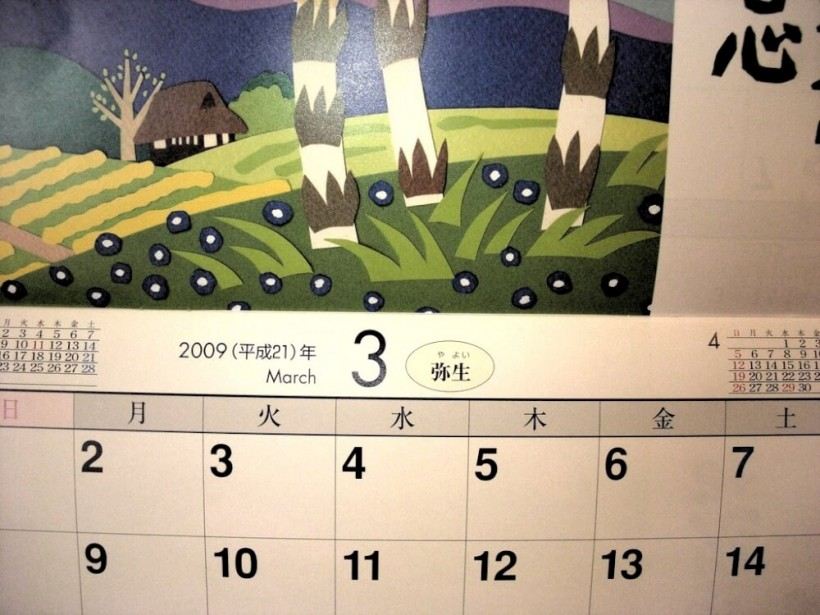# What is Heisei? – Understanding a Date in the Japanese Calendar

In Japan, there are currently two main calendar systems used, mainly differentiated by the year of reference. What you would already be familiar is the 西暦 (sei-reki – Anno Domini/Common Era) which uses a reference from around 2000 years ago. For example, at the time of writing, we are in 2014 A.D. (or C.E.).

The alternative year reference used in Japan is the 年号 (nen-gou – era name) which is pegged to the reign of the Emperors. The current era is the Heisei Era and this is the 26th year of the current Emperor’s reign, hence 2014 A.D. is the same year as Heisei 26. You may find some forms requiring such a field although leeway is often given even if you write the western year.

However, there exists a slight complication in the fact that the dates generally are not supposed to overlap and the reign of the Emperors do not start at a fixed day in the year. If you refer to the table below, you might already notice that the end of an era and the start of the next era fall on the same year (e.g. Taisho 1 and Meiji 45 both refer to 1912).

So which is the correct reference? Basically, all you have to do is to look at the start date of the era (2nd row), and that would be the day of transition. I.e. Any date before 30 July 1912 is Meiji 45, while anything after is Taisho 1.

 Meiji Era （明治） Taisho Era（大正） Showa Era　(昭和） Heisei Era　(平成) 8 Sep 1868 30 Jul 1912 25 Dec 1926 8 Jan 1989 Meiji 1 = 1868 Taisho 1 = 1912 Showa 1 = 1926 Heisei 1 = 1989 Meiji 2 = 1869 Taisho 2 = 1913 Showa 2 = 1927 Heisei 2 = 1990 Meiji 3 = 1870 Taisho 3 = 1914 Showa 3 = 1928 Heisei 3 = 1991 Meiji 4 = 1871 Taisho 4 = 1915 Showa 4 = 1929 Heisei 4 = 1992 Meiji 5 = 1872 Taisho 5 = 1916 Showa 5 = 1930 Heisei 5 = 1993 Meiji 6 = 1873 Taisho 6 = 1917 Showa 6 = 1931 Heisei 6 = 1994 Meiji 7 = 1874 Taisho 7 = 1918 Showa 7 = 1932 Heisei 7 = 1995 Meiji 8 = 1875 Taisho 8 = 1919 Showa 8 = 1933 Heisei 8 = 1996 Meiji 9 = 1876 Taisho 9 = 1920 Showa 9 = 1934 Heisei 9 = 1997 Meiji 10 = 1877 Taisho 10 = 1921 Showa 10 = 1935 Heisei 10 = 1998 Meiji 11 = 1878 Taisho 11 = 1922 Showa 11 = 1936 Heisei 11 = 1999 Meiji 12 = 1879 Taisho 12 = 1923 Showa 12 = 1937 Heisei 12 = 2000 Meiji 13 = 1880 Taisho 13 = 1924 Showa 13 = 1938 Heisei 13 = 2001 Meiji 14 = 1881 Taisho 14 = 1925 Showa 14 = 1939 Heisei 14 = 2002 Meiji 15 = 1882 Taisho 15 = 1926 Showa 15 = 1940 Heisei 15 = 2003 Meiji 16 = 1883 Showa 16 = 1941 Heisei 16 = 2004 Meiji 17 = 1884 Showa 17 = 1942 Heisei 17 = 2005 Meiji 18 = 1885 Showa 18 = 1943 Heisei 18 = 2006 Meiji 19 = 1886 Showa 19 = 1944 Heisei 19 = 2007 Meiji 20 = 1887 Showa 20 = 1945 Heisei 20 = 2008 Meiji 21 = 1888 Showa 21 = 1946 Heisei 21 = 2009 Meiji 22 = 1889 Showa 22 = 1947 Heisei 22 = 2010 Meiji 23 = 1890 Showa 23 = 1948 Heisei 23 = 2011 Meiji 24 = 1891 Showa 24 = 1949 Heisei 24 = 2012 Meiji 25 = 1892 Showa 25 = 1950 Heisei 25 = 2013 Meiji 26 = 1893 Showa 26 = 1951 Heisei 26 = 2014 Meiji 27 = 1894 Showa 27 = 1952 Meiji 28 = 1895 Showa 28 = 1953 Meiji 29 = 1896 Showa 29 = 1954 Meiji 30 = 1897 Showa 30 = 1955 Meiji 31 = 1898 Showa 31 = 1956 Meiji 32 = 1899 Showa 32 = 1957 Meiji 33 = 1900 Showa 33 = 1958 Meiji 34 = 1901 Showa 34 = 1959 Meiji 35 = 1902 Showa 35 = 1960 Meiji 36 = 1903 Showa 36 = 1961 Meiji 37 = 1904 Showa 37 = 1962 Meiji 38 = 1905 Showa 38 = 1963 Meiji 39 = 1906 Showa 39 = 1964 Meiji 40 = 1907 Showa 40 = 1965 Meiji 41 = 1908 Showa 41 = 1966 Meiji 42 = 1909 Showa 42 = 1967 Meiji 43 = 1910 Showa 43 = 1968 Meiji 44 = 1911 Showa 44 = 1969 Meiji 45 = 1912 Showa 45 = 1970 Showa 46 = 1971 Showa 47 = 1972 Showa 48 = 1973 Showa 49 = 1974 Showa 50 = 1975 Showa 51 = 1976 Showa 52 = 1977 Showa 53 = 1978 Showa 54 = 1979 Showa 55 = 1980 Showa 56 = 1981 Showa 57 = 1982 Showa 58 = 1983 Showa 59 = 1984 Showa 60 = 1985 Showa 61 = 1986 Showa 62 = 1987 Showa 63 = 1988 Showa 64 = 1989

If you are looking for dates of holidays in Japan instead, refer to this.

*Feature image credits: geraldford (CC license)